This blog has moved ! New look and new articles on www.naturelovesmath.com/en/

# Icosian : A graph theory game

 Neutral : for everyone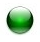Level 4 of the game "Icosian"
The Icosian 1 game is a graph theory game. It was invented in 1857 by Sir W.R.Hamilton (1805-1865), a great mathematician to whom we owe - among other things - a reformulation of mechanics' formalism which now bears his name, and quaternions (which we'll tackle in a future article).

In this post, we will focus on a flash version of this game, written by Neamar. More precisely, we will see the mathematical principles behind the game and a method for solving the last two levels.

If you don't know this game, don't read this article right now, but try the game beforehand !

You liked this game and would like to know a bit about graph theory ? You've been tearing your hairs out on the last two levels and would like to see a detailed solution ? Then here you go...

## Eulerian graphs

The first part of the game is easy enough and is only a warm-up. The goal is to find Eulerian cycles.
A graph is said to be "Eulerian" when it contains a Eulerian cycle : one can « run through » the graph from any vertex, passing by every edge and finish at the starting vertex. Note that every vertex is gone through at least one time and possibly more. In other words, we can trace the graph with a pencil without retracing edges or lifting the pencil from the paper.
It is forbidden to go more than once through an edge, or link two vertex that are not incident to a same edge. But it is possible to cross paths.

A fundamental theorem, by Leonard P.Euler (1707-1783) of course, assures us that a graph is Eulerian if and only if the number of edges incident to any vertex is even 2 (we say the vertex is of even degree).

So when a graph only has vertices of even degree, we can run it through from any vertex and back to it, passing by all the edges once ! It is the case of the first level, used as an example, and of levels 4, 6, 7 and 8 which are then very easy.Level 6

When a graph has even and odd degree vertices, it isn't Eulerian. But if it has exactly two vertices of odd degree (no more and no less), then one can start at one of them and pass by all edges once to finish at the second one. We then have a eulerian path. The graph is "nearly Eulerian" since it only needs one more edge linking the two vertices of odd degree to become Eulerian. It is the case of the levels 2, 3, 5 and 9 of the game. Those are easy provided one starts at a vertex of odd degree ! The level 9 can then appear difficult if one doesn't see these two preferred vertices to start from...Level 9

To conclude, determining if a graph has a eulerian path is very easy since one only needs to count the degrees of each vertex.

## Hamiltonian graphs

From level 10 (used as an example), rules change. Now the game asks the player to run through every vertex (once) instead of every edge (once). No problem at first sight, shouldn't be so hard ! Big mistake. We'll see that this problem can become arbitrarily difficult.

A graph is said to be "Hamiltonian" when it contains an hamiltonian cycle : one can « run through » the graph from any vertex and back, passing through every vertex. There can be more than one cycle and unfortunately there is no known theorem that characterizes Hamiltonian graphs, contrary to the Eulerian ones.
While not every graph of the first part of the game are Eulerian (some are "nearly Eulerian" because they only contain a eulerian path and no cycle as we've seen), every graph of the second part is Hamiltonian.

Levels 10 (Hamiltonian but not Eulerian graph), 11 and 12 are easy and their purpose is to familiarized oneself with hamiltonian cycles. Level 13 requires one to anticipate the way back but is still easy. Levels 14 and 15 aren't that much more difficult.Level 14

Level 16 is not difficult, 17 is just a little bit tricky and 18 only needs a few try. Then comes the 19 and it's a whole other story.
If it possibly can be solved by trial and error, it is way more complex than the other ones.

## Level 19Level 19

One way to find a hamiltonian cycle into a graph consists in trying every path... Possible for the preceding levels, it would be way too much time-consuming (without computer help) for this level or the next !
Instead, let's try to divide and rule, by cutting the graph into small sub-graphs and solving them in place of the big one. This method particularly fits level 19 since it is made of three identical sub-graphs, one at the top, one on the left and one on the right, looking like this :Part of level 19

Note that the right-hand sub-graph isn't connected by the same number of edges, because the creator of the game removed a vertex 3 from the original graph... it seems to make the problem harder, but we'll see that in fact it will make it easier for our method.

#### Step 1 :

Let's list some hamiltonian path in the sub-graph. No need to be exhaustive, a dozen possibility should be more than enough to find at least one solution of the general problem. We will see exactly how many solutions this (incomplete) list gives us. To be concise, we did not take account of the symmetrical path, which would imply even more solutions. The first path will appear in red, and in the next ones, different edges will be marked in blue, in order to differentiate them easily.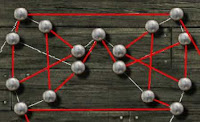Partial hamiltonian path n°1Partial hamiltonian path n°2Partial hamiltonian path n°3Partial hamiltonian path n°4Partial hamiltonian path n°5Partial hamiltonian path n°6Partial hamiltonian path n°7Partial hamiltonian path n°8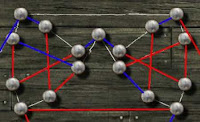Partial hamiltonian path n°9Partial hamiltonian path n°10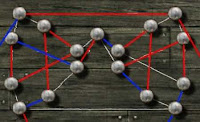Partial hamiltonian path n°11

#### Step 2 :

Let's sort it out. First, path number 5 is a cycle, so we can eliminate it right away. For the top sub-graph, the first four paths and the number 7, 9, 10 and 11 don't fit because then there wouldn't be any possibility left (in our - incomplete - list) for the right-hand sub-graph, because of the missing vertex. That leaves us with the 6 and 8 for the top sub-graph. We colored in green the edges that would necessarily be used in the hamiltonian cycle based on these sub-graphs' paths :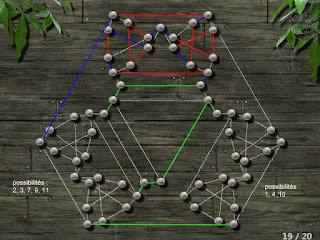Possibilities with top sub-graph number 6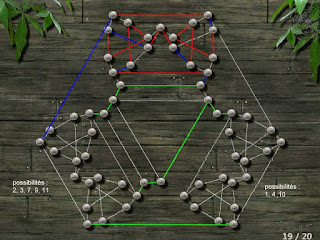Possibilities with top sub-graph number 8

These sub-graphs being quasi-identical, they offer the same combinatorial possibilities. Finally, we can enumerate the following solutions, expressed with three numbers (a,b,c) with a representing the top sub-graph, b the right-hand one and c the left-hand one :

(6,1,2); (6,1,3); (6,1,7); (6,1,9); (6,1,11); (6,4,2); (6,4,3); (6,4,7); (6,4,9); (6,4,11); (6,10,2); (6,10,3); (6,10,7); (6,10,9); (6,10,11);
(8,1,2); (8,1,3); (8,1,7); (8,1,9); (8,1,11); (8,4,2); (8,4,3); (8,4,7); (8,4,9); (8,4,11); (8,10,2); (8,10,3); (8,10,7); (8,10,9); (8,10,11);...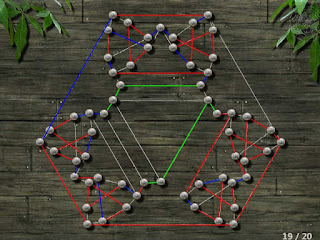Solution (6,10,11) given by Neamar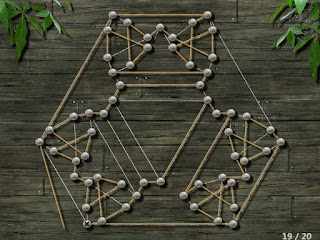Solution (6,1,7) given to me by a friend (thx Pierre !)

So there is a lot of different solutions, at least 30, and even more if we take into account the symmetrical paths (however be careful not to double this number because the graph isn't symmetrical !). Note that all the solutions differ only locally.

## Level 20Level 20

At first sight, this level seems way too complicated. If we try to solve it by trial and error, it could take a while. And there is no way to cut it up into sub-graphs... So what to do ?
Well, in fact it is possible to simplify the graph considerably, because it contains a lot of vertex of degree 2. These are a bargain because since every vertex will be in the cycle, the edges of those of degree 2 will necessarily be part of it too ! So we can temporarily eliminate these vertex and replace each of them with a new edge. So there we go, let's simplify :

#### Step 1 :

First we need to count the degrees of the vertices, to identify those we will be able to eliminate :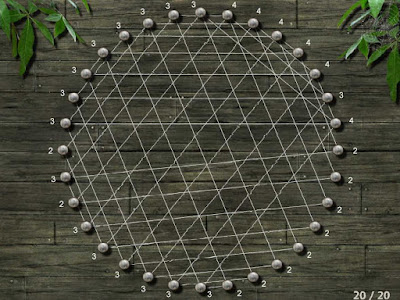There are 10 vertex of degree 2

#### Step 2 :

Mark the edges that will necessarily be part of the hamiltonian cycle :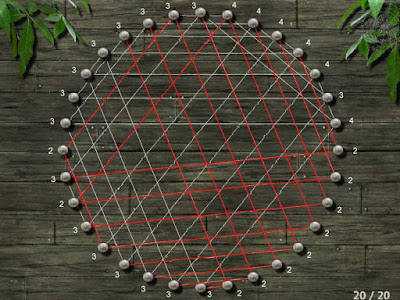Unavoidable edges

We can also eliminate some edges that will not be part of the cycle, and then decrease the degree of some vertices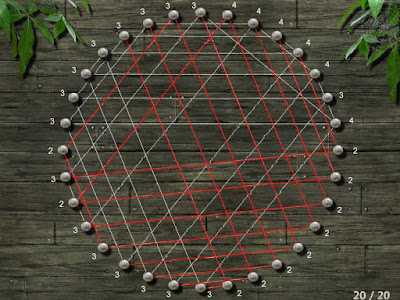Useless edges deleted

and repeatNew unavoidable edges

and repeat until there is no more vertex of degree 2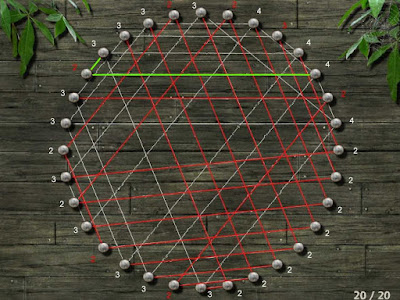New unavoidable edges

#### Step 3 :

Then we just have to replace each red path by a new edge (blue for example) and delete the corresponding vertices.

Step 4 :
Repeat Step 2 and 3 while it is possible.Simplification of the graph

And here is the result, the graph has been simplified as much as possible... Not so hard anymore, is it ?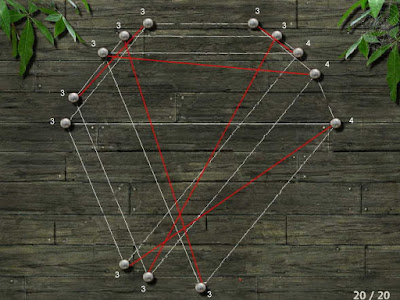Level 20 simplified

#### Step 5 :

Solve the simplified graph. It has exactly one solution :Solution of the simplified graph

#### Step 6 :

Copy the green edges into the red path of the graph to obtain the general solution. Notice that two edges are missing because the simplification was done several times over (in step 4), those are the blue edges.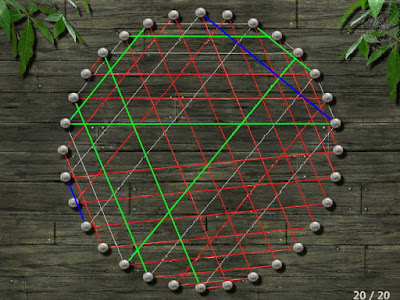General solution

Now we can trace the hamiltonian cycle in the level :

## Conclusion

So, easy are the problems of graph theory ? Certainly not ! Contrary to eulerian paths, find an hamiltonian cycle is a problem of class NP-complete, that is to say algorithmically difficult. Actually there is no algorithm that permits to find a hamiltonian cycle in any graph in a reasonable time (it's always possible to try all the paths but it is way too much time-consuming in general !). The levels 19 and 20 of this Icosian game are good examples of this difficulty : we had to use two completely different methods to solve them. Indeed, level 19 cannot be simplified like the 20 (we can only eliminate one vertex with this method...) and level 20 cannot be decomposed into sub-graphs like the 19.

So we had to use two different heuristics. A heuristic is like an quick algorithm, but one that doesn't guarantee a correct or optimal solution every time. It is possible to not find a solution at all using it (example : trying to simplify the graph of level 19 is useless).

To finish let's thank the creator of this game, Neamar, and the graphic designer Licoti, for what is certainly the most beautiful graph game on the internet !

#### Notes :

1. Originally, the game consisted in an exemplification of some symmetrical properties of the icosahedron (and of the dodecahedron, the two having the same symmetries), hence the term "Icosian".

2. This theorem concerns connected graphs, that is to say "made of one piece". We will only consider connected graph here.

3. Neamar used Hypohamiltonian graphs (having the property of becoming an Hamiltonian graph when any vertex is removed). It is the case of the graphs from levels 15, 16 and 19 which thus miss a vertex.0 Responses to "Icosian : A graph theory game"